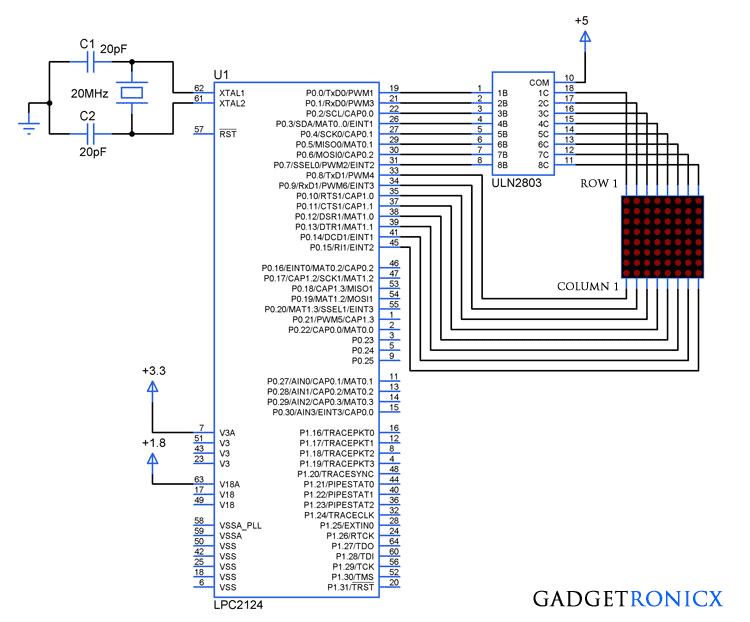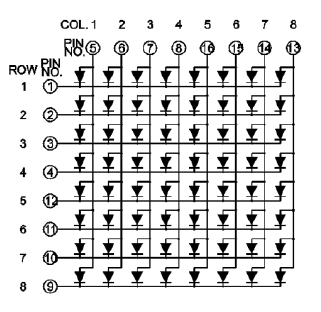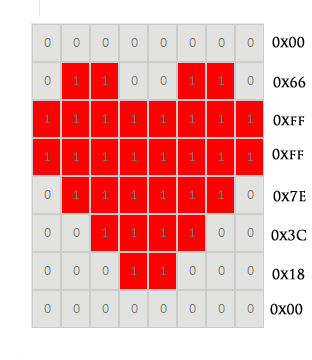# 8×8 LED dot matrix interfacing with ARM MicrocontrollerDot matrix interface with LPC2124

LED’s have a wide range of lighting applications in our daily life and surprisingly it also can be used as a display when interconnected together. LED dot matrix is nothing but collection of LED’s interconnected together which can be lit by accessing through rows and columns. This dot matrix is used in banks, lifts, buses and much more where displaying of information is necessary. This tutorial demonstrates dot matrix interfacing with arm7 series microcontrollers.

## 8×8 DOT MATRIX:Common anode LED matrix

The above diagram shows the connection configuration of 8×8 LED dot matrix where each row and column consists of 8 LED’s totally. The above figure shows the common Anode configuration where the LED’s are sourced through the columns from the Microcontroller pins and sinked through the rows to the pins. The Switching of the LED’s should be faster to form a pattern in the dot matrix display.

## ULN2803:

The sinking current from the LED’s can damage the pins of the Microcontroller since the current sinking capability of the controller is very less. So a IC ULN2803 was used here which is capable of sinking high currents from the rows of the dot matrix. Each pins of the ULN2803 can be switched by applying the high logic to the input pins of the ULN2803 from the pins of LPC2124. Read Complete working of ULN2803 for better understanding.

## STEPS TO DISPLAY A CHARACTER IN DOT MATRIX:

• Make the pins of each row high one by one, once high signal is applied to the ULN2803 input it will sink the currents of LED in that row.
• Activate column pins for your pattern or character for each rows.
• Use very small delay in the matter of milli seconds between each switching, this will make the dot matrix to display a your desired pattern or character.

## TO DISPLAY A HEART SYMBOL IN DOT MATRIX:Heart Symbol display

This tutorial demonstrates displaying a heart symbol in the LED dot matrix. As you can see in the above diagram the dot matrix consists of 8 rows and columns with a heart drawn over it. The 1 represents the LED’s which need to switched ON to display the pattern whereas  0 represents the LED in off state. The respective values for each column of each row activation was given, all you need to do is place the values in array and assign it to the pins of the Controller.

## CODE:

This code was built using Keil uVision 4.

```#include<lpc21xx.h>
unsigned char row_val={0x01,0x02,0x04,0x08,0x10,0x20,0x40,0x80};
unsigned char col_val={0x00,0x66,0xff,0xff,0x7e,0x3c,0x18,0x00};
void delay(void);
int a;
int main(void)
{
PINSEL0=0;
PINSEL1=0;
IODIR0=0x0000ffff;
while(1)
{
for(a=0;a<=7;a++)
{
IOSET0|=(row_val[a]<<0)|(col_val[a]<<8);
delay();
IOCLR0|=(row_val[a]<<0)|(col_val[a]<<8);
}
delay();
}
}

void delay(void)
{
unsigned int i;
for(i=0;i<=2000;i++);
}```

.

## JLCPCB - Only \$2 for PCB Prototype (Any Color)

Up to \$20 shipping discount on first order now: https://jlcpcb.com/quote